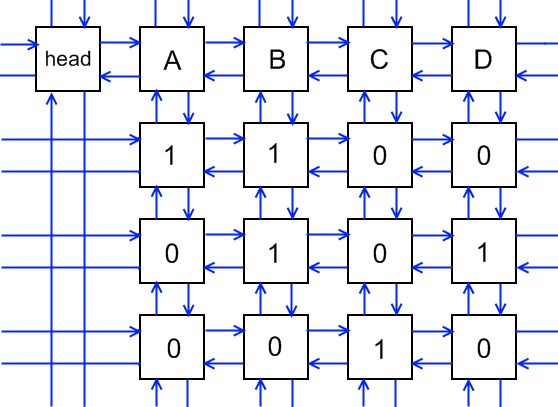o
osc_y8yehimr### 算法描述

1. 选择矩阵中一列$c$，考虑用某该列为1的行来覆盖这一列。
2. 枚举该列为1的行，比如$r$行。
3. 删除因为此次决策被覆盖掉的列、行，以及因为此次决策而不可能选取的行。
4. 递归搜索，从第1条开始。
5. 回溯，恢复本次决策删掉的所有行和列。

Arxiv Paper

## 数独问题

1. 每个格子必须且仅能填1～9中的一个数。
2. 每一行上1～9每个数必须且仅用一次。
3. 每一列上1～9每个数必须且仅用一次。
4. 每个子宫格内1～9每个数必须且仅用一次。

class Base(object):
def __init__(self, id):
self.id = id
self.l = self
self.r = self
self.u = self
self.d = self

def __init__(self):

class Cell(Base):
def __init__(self, x, y, c=None):
super().__init__(id=('cell', x, y))
if c:
self.c = c
self.u = c.u
self.d = c
self.u.d = self
self.d.u = self
self.c.s += 1

class Coln(Base):
def __init__(self, c):
super().__init__(id=('coln', c))
self.s = 0

def _cover(self, c):
c.r.l = c.l
c.l.r = c.r
i = c.d
while i != c:
j = i.r
while j != i:
j.d.u = j.u
j.u.d = j.d
j.c.s -= 1
j = j.r
i = i.d

def _uncover(self, c):
i = c.u
while i != c:
j = i.l
while j != i:
j.c.s += 1
j.u.d = j
j.d.u = j
j = j.l
i = i.u
c.l.r = c
c.r.l = c

def _choose_col(self):
c, s = None, float('inf')
i = self.h.r
while i != self.h:
if s > i.s:
c, s = i, i.s
i = i.r
return c

def _search(self, s):
if self.fall or len(self.ans) == 0:
if self.h == self.h.r:
self.ans.append(s[:])
else:
c = self._choose_col()
self._cover(c)
i = c.d
while i != c:
s.append(i.id)
j = i.r
while j != i:
self._cover(j.c)
j = j.r
self._search(s)
j = i.l
while j != i:
self._uncover(j.c)
j = j.l
i = i.d
self._uncover(c)

def run(self, find_all=False):
self.ans = []
self.fall = find_all
if self.h != self.h.r:
self._search([])
return self.ans

@classmethod
def from_sudoku(cls, grid):
n = len(grid)
m = int(n**0.5)
a = []
for i in range(4*n*n):
c = Coln(i)
c.r = h
c.l = h.l
c.l.r = c
c.r.l = c
a.append(c)
pos = lambda x, y, k: x * n + y
row = lambda x, y, k: x * n + k + n*n
col = lambda x, y, k: y * n + k + n*n*2
box = lambda x, y, k: ((x // m) * m + (y // m)) * n + k + n*n*3
idx = lambda x, y, k: (x * n + y) * n + k
a.l, b.l, c.l, d.l = d, a, b, c
a.r, b.r, c.r, d.r = b, c, d, a
def create(x, y, k):
p = Cell(x=idx(x, y, k), y=pos(x, y, k), c=a[pos(x, y, k)])
r = Cell(x=idx(x, y, k), y=row(x, y, k), c=a[row(x, y, k)])
c = Cell(x=idx(x, y, k), y=col(x, y, k), c=a[col(x, y, k)])
b = Cell(x=idx(x, y, k), y=box(x, y, k), c=a[box(x, y, k)])
for i in range(n):
for j in range(n):
if grid[i][j] == 0:
for k in range(n):
create(i, j, k)
else:
create(i, j, grid[i][j]-1)
return cls(h)

def soduku(grid):
grid = [i[:] for i in grid]
n = len(grid)
a = d.run()
for i in a:
r = i
k = r % n
y = (r // n) % n
x = ((r // n) // n) % n
grid[x][y] = k + 1
return grid

def nextline():
s = input().strip()
if len(s) == 0:
return nextline()
else:
return s

def soduko9():
def input_grid():
grid = []
for i in range(9):
grid.append([])
for j in nextline():
grid[-1].append(int(j))
return grid

def output_grid(grid):
for i in grid:
for j in i:
print(j, end='')
print("")

cas = int(input())
for c in range(cas):
output_grid(soduku(input_grid()))

def soduko16():
def input_grid():
grid = []
for i in range(16):
grid.append([])
for j in nextline():
grid[-1].append(ord(j)-ord('A')+1 if j != '-' else 0)
return grid

def output_grid(grid):
for i in grid:
for j in i:
print(chr(ord('A')+j-1), end='')
print("")
print("")

while True:
try:
output_grid(soduku(input_grid()))
except EOFError:
exit(0)

if __name__ == '__main__':
# soduko9()
# soduko16()


## 参考资料

o

### osc_y8yehimr

2013/11/03
3.3K
6

Huginn 是雅虎开发的一个系统，可以帮你执行自动化的在线任务。可以阅读网页,关注事件,并采取相应操作。Huginn 通过一个直观的事件流图来展示各种操作和事件。通过在你自己的服务器上的管道加...

2013/03/15
1.7W
0
PHP web 服务器--YACS

YACS 是一个强大的 PHP 脚本，可以让你维护一个动态的 Web 服务器。 特性： - Runs on your own server, or on a shared web site - Post articles with web forms, by e-mail, or remotely ......

2013/03/18
847
0

PS320 V0.12 版本为开源版本 本程序最初想解决自己管理服务器问题，当初因客户不对外开放端口，导致管理工作量大，速度慢且不稳定，每次管理都去现场处理。 本程序提供端口映射功能，可以直接...

2013/06/03
2.1K
0
Android防火墙软件--imiPhonewall

imiyoo
2013/06/07
1K
0

Linux拜拜！微软给WSL加入GPU支持，Windows终于迎来命令行包管理工具

05/25
0
0
GraphQL

2分钟前
0
0

javail
25分钟前
5
0
MySql查询所有字段不为空值的数据及Mybatis的#号和\$符的区别引起的问题

1.MySql查询所有字段不为空值的数据 搜了一上午搜不到，最后用Mybatis的foreach标签，先查询出表字段， SELECT COLUMN_NAMEFROM INFORMATION_SCHEMA.ColumnsWHERE table_name='lltest'...

25分钟前
28
0

WebRTC中文社区是一个为大家解决在使用WebRTC当中遇到问题所建立的社区，欢迎更多学习和使用WebRTC的人加入进来，一起建设。 视频录制 在之前的文章里我们提到过视频录制的两种方式：客户端录...

32分钟前
13
0